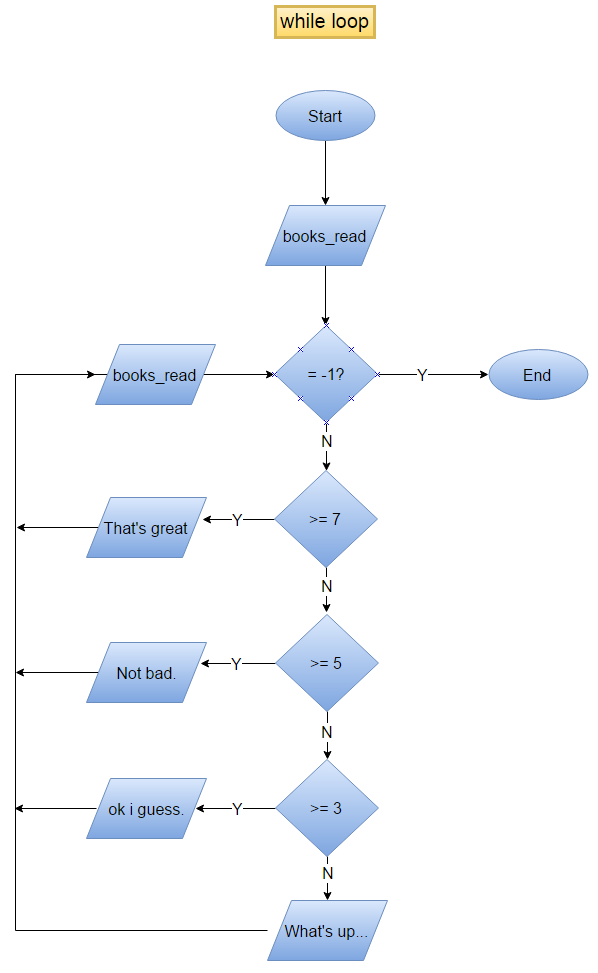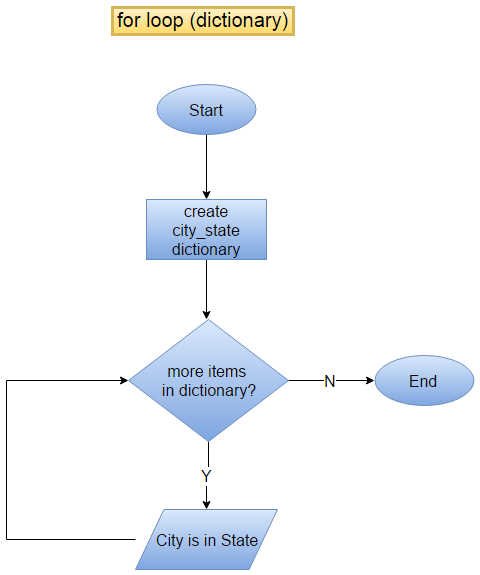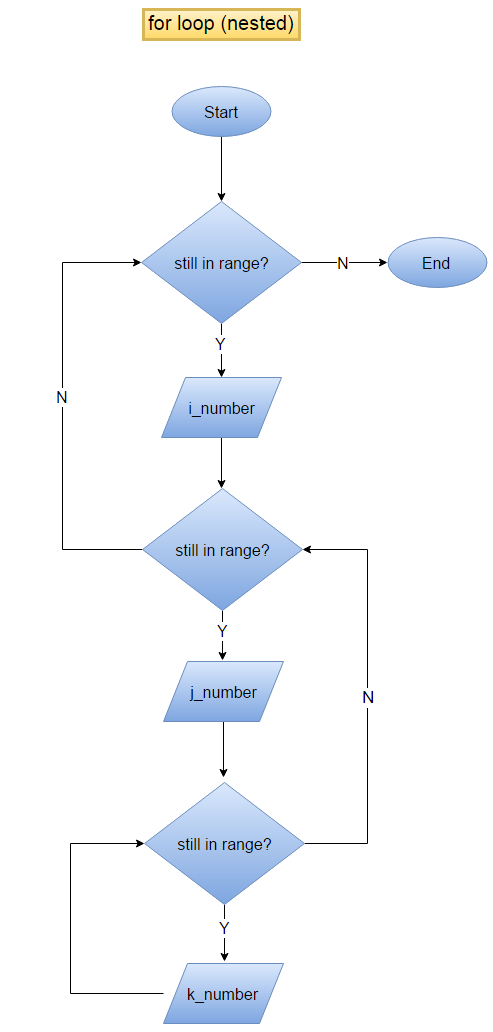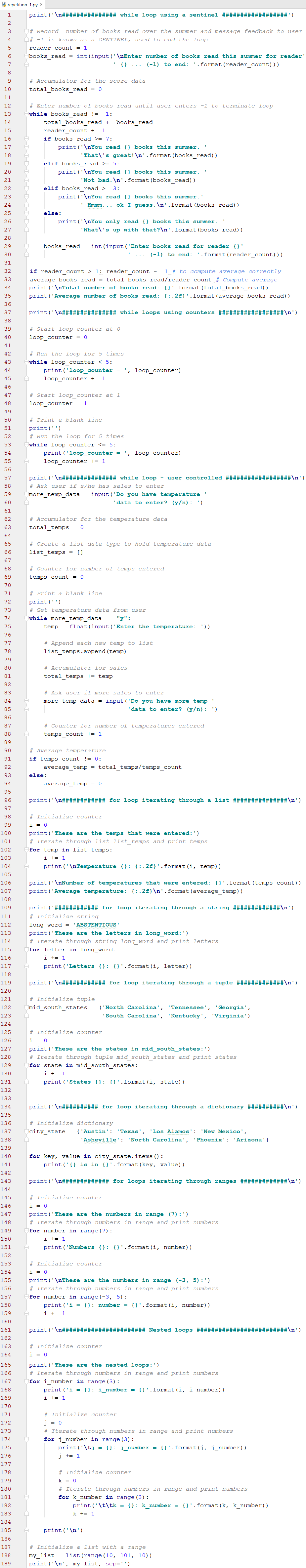COSC 1336 | PyThonRepetition

Foreword

Repetition is the third of the three programming control structures. Programs often need to perform the same set of steps over and over, that is when we use a repetition control structure. There are a variety of repetition options available to Python developers. A few of the alternatives are described below.

The following five repetition structures are explained in this chapter:

• While loop
• For loop (in a sequence)
• For loop (in a dictionary)
• For loop (in a range)
• Nested Loops

While Loop

The while loop is a general purpose repetition construct used in Python and essentially all other modern programming languages. A while loop can be user-controlled, counter-controlled, or controlled via external input such as from a file. The code section below includes examples of user-controlled and counter-controlled loops. A while loop working with file data is covered in the 'File I/O' chapter.For Loop (in a sequence)

A for loop is an iterator and can be used to step through any ordered sequence or other type of iterable object. Lists, strings, and tuples are common sequence types used with for loops. Examples of each are included in the code below. We will see more of lists, strings, and tuples in the 'Data Type - Sequences' chapter.For Loop (in a dictionary)

Even though a dictionary is a mapping data type and not a sequence, it is still iterable and therefore can be used with a for loop. In the code below, a dictionary named city_state is created and a for loop is used to iterate through the contents. Notice on line 140 that the items() method of the dictionary class is used to populate the keys and values. We will see more on the dictionary in the 'Data Type - Mappings' chapter.For Loop (in a range)

The official Python library reference includes 'range' as a sequence data type but the official Python language reference does not. A range does support iteration and can therefore be used with a for loop. In some locations of the documentation, range is identified as a built-in function and in others it is considered an immutable sequence type. In this course, I will defer to the Python language reference which does not include range as a data type.

Notice that multiple examples using ranges can be found on lines 149-185. When we write range(a), the function will return integer numbers from 0 to a-1. So, range(8) will iterate 0-7 and range(11) will iterate 0-10. On line 157, range(-3, 5) is used to return the numbers -3 through 5-1. Finally, we can supply a third argument such as (5, 50, 5) which will return the numbers from 5 to 50-1 but using 5 as the step value. Code this step example and observe the values returned.Nested Loops

In the last section of the code a nested loop example is provided. A key point to understand about nested loops is that the inner loop iterates N number of times for every one time the outer loop iterates. This can be observed in the output produced from lines 167-185. Note in the output that i = 0 (i is the outer loop counter) while j moves from 0 to 2 and that k moves from 0 to 2 for each value of j from 0 to 2.

Construct your own example and experiment with the values to understand more thoroughly. Another important note to consider is the number of times the inner most statements will be executed. The inner most statements in the example are lines 182 and 183. The answer to the question can be computed by multiplying the number of times each of the loops will be executed. In the case of the example the answer is: 3 x 3 x 3 = 27.Multiple repetition control structures are demonstrated in the code listing below. Be sure to code the example and follow the output to ensure understanding.Here's the output.